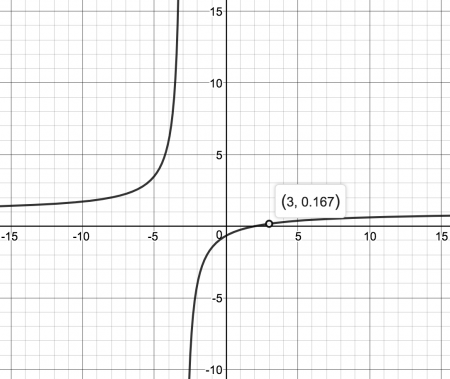If you’re a faculty member, you can probably tell me all about the first time an instructional designer said to you “You can’t use the word ‘understand’ in a learning objective.” And it probably made you mad.

The oft-cited reason to avoid “understand” is because “You can’t measure it.” And this is where I’d like to take issue with Instructional Design 101.  Can you always measure an “understand” learning objective? No. Can you sometimes measure an “understand” learning objective? Yes. And it really comes down to the definition you use.

understand: mental comprehension of a topic

understand: demonstrated comprehension of a topic

In particular, if “understand” is used in the “demonstrated comprehension” form, we can measure a collection of skills that, taken together, show a broader understanding of the topic. It’s the difference between measuring the health of the forest or measuring each individual tree.Courses with a large body of objective knowledge and skills to be learned may have hundreds of granular learning objectives (think about courses like math, chemistry, anatomy, Spanish, and immunology). But if we only focus on granular objectives, we are focusing only on the google-able information and not on whether the student can demonstrate they have a broad understanding or deeper comprehension. In these types of subjects, I think that “understand” is being used in what I would call a “collector learning objective (or collector LO for short).

Okay, for instructional designers, that sounds pretty heretical. Let me prove it to you with an example from math.

Learning objective: Understand the relationship between a rational function and its graph.

As a math instructor, I have a pretty good idea of what I am hoping to get out of this objective on an assessment. And I’d be willing to bet money that any math instructor who teaches rational functions would come up with at least 75% of the same common list of sub-objectives we hope students can demonstrate as part of this comprehension-level “understand” objective from above:

• Determine the zeros of the graph of the rational function.
• Identify the y-intercept of the graph of the rational function.
• Determine the vertical asymptotes of the graph of the rational function (if any).
• Determine the horizontal asymptote of the graph of the rational function (if any).
• Determine whether the graph of the rational function has any holes, and where they are located.
• Determine the domain of the rational function.
• List the intervals where the graph of the rational function is increasing or decreasing.
• Estimate where the graph of the rational function is concave up or concave down.

This is where an assessment persons always chimes in: “Why not just write every learning sub-objective and assess each one individually?” Because then we are leading the student to specific answers instead of letting them explore the space and demonstrate what they know. Plus, I can assess the “understand” objective. I do it with a problem like this:

For the rational function  f(x)=(2x-1)/(x+4), tell me at least 8 properties that relate the equation to the graph.

Will I get statements representative of all 8 sub-objectives from every student? No, but I’ll probably get a representation of 75% of them for any student with reasonable competency.

I consider this learning objective “Understand the relationship between a rational function and its graph.” to be a collector learning objective, and we could write many other assessment questions that would also provide measurement:

Problem: Determine all the intercepts of a rational function.

Assesses these sub-objectives:

• Determine the zeros of the graph of the rational function.
• Identify the y-intercept of the graph of the rational function.

More broadly provides evidence for this collector LO:

• Understand the relationship between a rational function and its graph.

Problem: Determine all the asymptotes of a rational function.

Assesses these sub-objectives:

• Determine the vertical asymptotes of the graph of the rational function (if any).
• Determine the horizontal asymptote of the graph of the rational function (if any).
• Determine whether the graph of the rational function has any holes, and where they are located.

More broadly provides evidence for this collector LO:

• Understand the relationship between a rational function and its graph.

Problem: Determine the equation of a possible rational function given the graph below.Assesses these sub-objectives:

• Determine the zeros of the graph of the rational function.
• Identify the y-intercept of the graph of the rational function.
• Determine the vertical asymptotes of the graph of the rational function (if any).
• Determine the horizontal asymptote of the graph of the rational function (if any).
• Determine whether the graph of the rational function has any holes, and where they are located.
• Determine the domain of the rational function.

More broadly assesses this collector LO:

• Understand the relationship between a rational function and its graph.

The collector learning objective describes the actual skill I want students to walk away from the class with. An ability to show general competency about rational function equations and graphs without specific prompts about what to say or do.’

One of the biggest problems with telling faculty they “can’t use understand” is that the conversation starts with one person telling the other that they are wrong, when in fact, they might not be.

Simply the existence of the collector learning objective (and the word understand) tells me this is a big-picture skill. I’m looking for a forest, not the trees.

If you’re an instructional designer, try poking at the “understand LO” in a different way – seek to understand the point of the objective yourself (punny, huh?) and ask the faculty to tell you whether they have an assessment strategy in mind. Because they just might.